Email : info@epmstrategy.com | Call Us Now : 479-321-3977
:: Home :: Sitemap ::

# Power BI Understanding the Basics of Using Variables in DAX

BY: David Rohlfs

Introduction

One of the most difficult aspects of using DAX when you are still learning is understanding the syntax of what you are writing. Fortunately, variables can help to solve this issue. While variables have many purposes and can be very useful for writing complex DAX equations, this blog will focus on the basic idea of what variables in DAX are and why they are useful.

This blog will assume that you have a basic understanding of what DAX functions and formulas are and how they are built.

In this blog we will go through when to use variables, some common problems that you might encounter, and a short walkthrough explaining how to write a variable equation in DAX.

When to use it

The biggest advantage of using variables in DAX is the simplicity of the syntax. Normally when you write a DAX equation your goal is to use the least number of measures to accomplish the equation you want. To do this you create a measure that looks like this: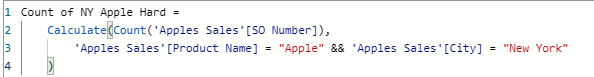You can still read this equation and understand it easily, but even with this small dataset the equation looks messy.

What Variables do is allow you to take each part of that measure and break them into their own equations. For the above example that could look like this: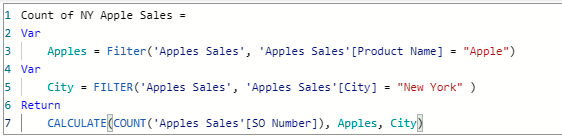Now you can understand how the filters are shown in the final equation without needing to check for any stray parenthesis or sub-functions.

The advantages of using the variable statement are:

• Simple to understand and read
• Easier to debug
• Improves performance
• Can be used for simple or complex equations

The way that a Variables statement works is by splitting up an equation into Variables and Results. The variables are the subsets of an equation, while the result is using those variables to determine the final equation. For instance, in this equation we are using variables to filter for products that are apples and New York as the city. In our result we are counting the number of orders that accept these filters to return us a numeric value.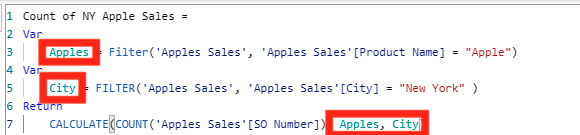In this screenshot you can see that we are only needing to focus on the Return function while the VAR functions are supporting your statement and developing the supporting equations. This is so useful when you need to develop DAX equations that have any number of sub-functions (Functions inside other functions).

You can use contingent equations in the RETURN portion of your equation. For instance, in the EPM Blog over Conditional Formatting Using DAX, we went through an example doing exactly this.

Using variables in your equations can help to improve report performance.

Common Problems

Overusing Calculate Function

A problem that has been found while using variables in DAX is when you use the Calculate () function in the Return section of your equation. Because your variables are listed in the same equation, the equation can often be overcomplicated and return incorrect results if you use a calculate function in the return portion of your equation. To fix this problem you can usually use a similar function or just take out the calculate function from your return. Doing this will allow for your data to develop properly and not return incorrect values. This is also good practice for all parts of your equation. An advantage of using variables in DAX functions is creating simple syntax. In addition, you should always look for other ways to simplify your equations.

Walkthrough

This walkthrough will be showing an example of a simple variable function in DAX and explaining the steps to build it. Because you will not have the same dataset as this, your fields and equation depth will likely be different, but this is meant to be a start to understanding variables in DAX.

First you are going to want to create a measure.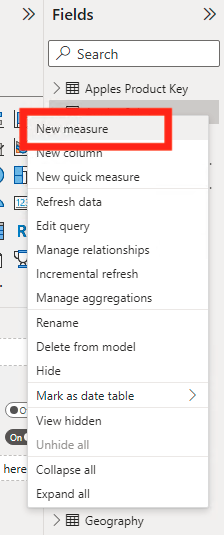Now you can type your measure name and equals sign. Remember that how you name your measure is how other people will know what you are trying to accomplish. When the goal of using variables is to simplify the syntax for an equation, you should remember to make every part of the equation easy to understand by others.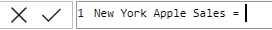To go to the next line of your formula, click Shift + Enter. The you can write out your first VAR statement. To do this you will type:

VAR Subtitle = Equation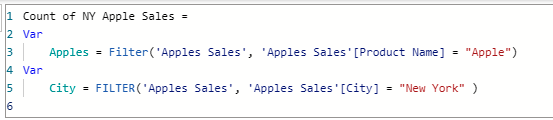You need to create a subtitle for your variables so that you can easily plug them into your return equation or subsequent VAR equations.

In your VAR equation you are just creating the equation for that variable. This means that you can go as low as possible to create your variables. For instance, if I only want to use sales that are greater than \$100, I might write my first variable to reflect that and develop my other variables using that.

Once you have written all your VAR statements, you can write your Return Statement. For this example, we will only be using a simple equation to get a final count on all the rows we have remaining.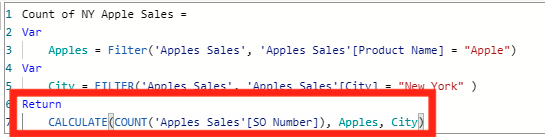The Return statement can be as short as the statement that I created in this walkthrough, but you can still plug in larger equations that use your above variables to develop solutions. This is an example from Conditional Formatting Using DAX that does this. It uses a Switch () function and searches through the variables for these values to give me my solution.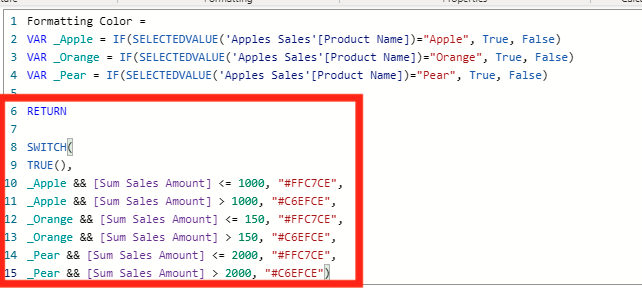Conclusion

As you grow in your understanding of DAX, variables become a much more important part of understanding complicated equations and measures. Although this blog uses a very simple dataset to explain how a variable works, it helps to depict the capabilities that this statement has when you create DAX equations.

### 3 Responses to “ Power BI Understanding the Basics of Using Variables in DAX ”

1. […] Now we get to build our equation. Because this is a longer and more complex equation, I am going to use variables in my equation. To learn how these work with DAX, you can read EPM Strategy’s blog on DAX Variables. […]

2. […] Power BI Understanding the Basics of Using Variables in DAX : :: Welcome To EPM Strategy :: […]

3. […] Power BI Understanding the Basics of Using Variables in DAX : :: Welcome To EPM Strategy :: […]